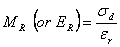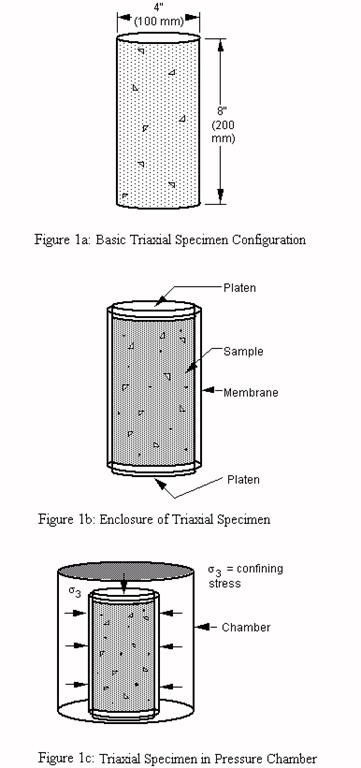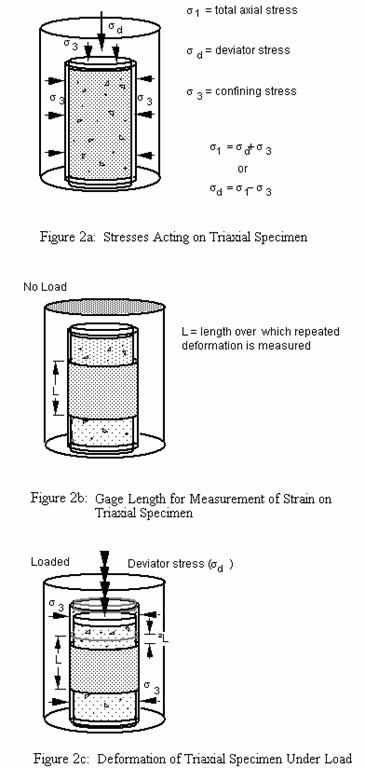# Triaxial Test

In a triaxial resilient modulus test a repeated axial cyclic stress of fixed magnitude, load duration and cyclic duration is applied to a cylindrical test specimen. While the specimen is subjected to this dynamic cyclic stress, it is also subjected to a static confining stress provided by a triaxial pressure chamber. The total resilient (recoverable) axial deformation response of the specimen is measured and used to calculate the resilient modulus using the following equation:## Test Description

The following is a basic outline of the triaxial test procedure:

1. The specimen is a cylindrical sample normally 100 mm (4 in.) in diameter by 200 mm (8 in.) high (Figure 1a).  The sample is generally compacted in the laboratory; however, undisturbed samples are best if available (which is rare).
2. The specimen is enclosed vertically by a thin “rubber” membrane and on both ends by rigid surfaces (platens) as sketched in Figure 1b.
3. The sample is placed in a pressure chamber and a confining pressure is applied (s3) as sketched in Figure 1c.
4. The deviator stress is the axial stress applied by the testing apparatus (s1) minus the confining stress (s3). In other words, the deviator stress is the repeated stress applied to the sample.  These stresses are further illustrated in Figure 2a.
5. The resulting strains are calculated over a gauge length, which is designated by “L” (refer to Figure 2b).
6. Basically, the initial condition of the sample is unloaded (no induced stress). When the deviator stress is applied, the sample deforms, changing in length as shown in Figure 2c.  This change in sample length is directly proportional to the stiffness.Figure 1: Triaxial specimen setup.

—-Figure 2: Triaxial test.

## Standard Test Methods

• AASHTO T 292: Resilient Modulus of Subgrade Soils and Untreated Base/Subbase Materials